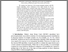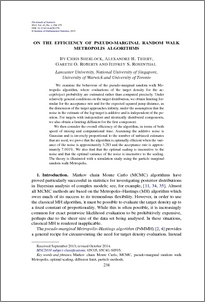# On the efficiency of pseudo-marginal random walk Metropolis algorithms

Sherlock, Christopher and Thiery, Alex and Roberts, Gareth and Rosenthal, Jeffrey (2015) On the efficiency of pseudo-marginal random walk Metropolis algorithms. Annals of Statistics, 43 (1). pp. 238-275. ISSN 0090-5364Preview
PDF (AOS1278)
AOS1278.pdf - Published Version

## Abstract

We examine the behaviour of the pseudo-marginal random walk Metropolis algorithm, where evaluations of the target density for the accept/reject probability are estimated rather than computed precisely. Under relatively general conditions on the target distribution, we obtain limiting formulae for the acceptance rate and for the expected squared jump distance, as the dimension of the target approaches infinity, under the assumption that the noise in the estimate of the log-target is additive and is independent of the position. For targets with independent and identically distributed components, we also obtain a limiting diffusion for the first component. We then consider the overall efficiency of the algorithm, in terms of both speed of mixing and computational time. Assuming the additive noise is Gaussian and is inversely proportional to the number of unbiased estimates that are used, we prove that the algorithm is optimally efficient when the variance of the noise is approximately 3.3 and the acceptance rate is approximately 7.0%. We also find that the optimal scaling is insensitive to the noise and that the optimal variance of the noise is insensitive to the scaling. The theory is illustrated with a simulation study using the particle random walk Metropolis.

Item Type:
Journal Article
Journal or Publication Title:
Annals of Statistics
Uncontrolled Keywords:
/dk/atira/pure/subjectarea/asjc/1800/1804
Subjects:
Departments:
ID Code:
72384
Deposited By:
Deposited On:
07 Jan 2015 08:55
Refereed?:
Yes
Published?:
Published